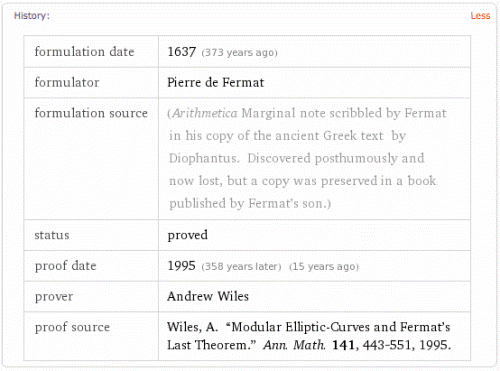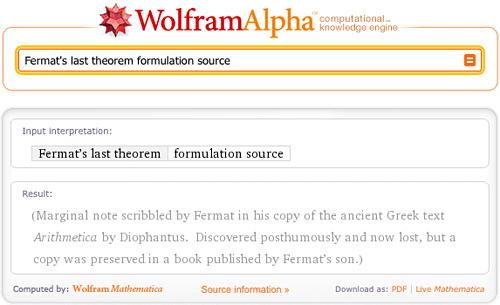# Math and Physics Results We Can Name

May 4, 2010 —Comments Off

As we all know by now, Wolfram|Alpha is a computational knowledge engine. That means not only should it be able to do computations on a wide variety of topics, but also that it needs detailed knowledge of the names and salient properties of a wide variety of entities that are commonly encountered in human inquiry and discourse.

This is obvious in the case of classes of objects that fit neatly into curated data collections, such as mathematical surfaces (e.g., Möbius strip), countries of the world (e.g., New Zealand), chemicals (e.g., caffeine), and so forth.

What is perhaps slightly less obvious is just how much knowledge needs to be encoded to have a reasonable “understanding” of almost any named result in math and the sciences. For example, most people (including non-mathematicians) have heard of Fermat’s last theorem and therefore would rightly expect Wolfram|Alpha to be able to say something sensible about it.  And as one of my other hats involves writing the online encyclopedia of math known as MathWorld, which is hosted and sponsored by Wolfram Research, putting this information into Wolfram|Alpha naturally fell to me. So, for the past several months, I have been attempting to gradually build up Wolfram|Alpha’s knowledge base on named results in math and physics.

The screenshot below shows what Wolfram|Alpha now returns for Fermat’s last theorem:As you can see, Wolfram|Alpha begins by giving you the standard name for the result in question, followed by a clearly worded (or at least as clearly worded as could be managed in the marginal space available 😉 ) plain English statement of the result. Next, at least in cases where it is possible to do so, a mathematically precise “formal statement” of the result is given. This is followed by any common alternate names the result might have, a listing of historical information, and finally an enumeration of prizes associated with it (where relevant).

There is also a “More” button in the History section (or “pod” as we like to call them), which displays much more detailed information, including original literature citations, information about when the result was first proposed and proved, who proposed and proved it, and so forth.Now, one of the important ideas behind Wolfram|Alpha is that everything should be computable. And while there’s surely much more that can (and will) be done in this direction, you can already ask questions like “who proved Fermat’s last theorem”, “who formulated FLT”, “Fermat’s last theorem proof year”, and “Fermat’s last theorem formulation source” and have them sensibly answered:As of this writing, Wolfram|Alpha knows 196 named mathematical results, and this number is growing weekly. Some other ones you might care to try include Bieberbach conjecture, Gödel’s incompleteness theorem, prime number theorem, and 3SAT. If you’re curious about the whole list, trying querying for famous math problems.

Similar results are also available for named results in physics. For example, you can try things like Kepler’s first law:

The physics results include associated formulas where relevant, in addition to the other properties, and will someday be more tightly integrated with Wolfram|Alpha’s formula framework (see for example, Kepler’s third law to see both the “formula” and “physics problem” interpretations of this input—note in particular the “Assuming” at the top, which lets you toggle between the two).

As of this writing, Wolfram|Alpha knows 45 named physics results, a number that is also growing weekly. Some other ones you might care to try include the EPR paradox, Schrödinger’s cat, and the Biot-Savart law. To see a full list, try famous physics problems.

Wolfram|Alpha’s collection of named problems also includes interesting or useful class tagging in additional to just entities. For example, you can ask for things like physical effects, Newton’s laws, NP problems, prize problems, and so on.Besides closer integration with the Wolfram|Alpha formula framework mentioned previously, additional enhancements coming soon include entrained birth/death information and time lines for people involved. Interlinking will eventually also work the other way, with queries for people being able to also mention problems they are associated with—for example, Albert Einstein‘s association with the photoelectric effect.

There are hundreds if not thousands of additional math and physics results that could be added to Wolfram|Alpha. So keep coming back often if you’re interested to see what new things we can tell you about and compute with for you. Of course, there are thousands if not millions of other interesting results in the sciences that are not fortunate enough to be blessed with a common moniker. That’s a more difficult problem. For now at least, as the Austrian philosopher Ludwig Wittgenstein noted, “That which we cannot name we must pass over in silence.”

Love this. Extremely useful… and starting to show more and more of the power of an informal interface mapping to formal knowledge with discrete output.

Now, I’m not so certain ol’ LWs quote is put in the proper context…. especially considering the point of Wolfram|Alpha…. Wolfram|Alpha suggests we don’t have to PASS OVER IN SILENCE what we cannot name. Instead let’s start somewhere and COMPUTE… perhaps we’ll find new stuff that we can then name for future reference…. just a thought.

Posted by Russ May 4, 2010 at 6:27 pm

Nice, but at this time too incomplete to be useful …

If WolframAlpha recognized “Poincare conjecture”

it was puzzled by the following entries:

Cauchy Schwarz inequality
Thales theorem
Parseval’s theorem
law of great numbers

Sylvain

Posted by Sylvain Flamant May 4, 2010 at 7:13 pm

Finally, thank you Eric! Now, lets see MMA 8 directly draw from your massive math dict instead of the notebooks. Btw, will you be helping put proofs as well for thats just as important into W/A. Keep it up.

Posted by Andrew Meit May 4, 2010 at 7:47 pm

Shouldn’t the status read “proven” rather than “proved”? I believe “proved” is the past-tense active verb, whereas “proven” is the past-tense passive participle, which is what you want.

Otherwise, awesome!

Posted by whatgoodisaroad May 4, 2010 at 8:23 pm

Very neato!

http://www.wolframalpha.com/input/?i=mathematical+theorems
shows them all.

So, when will we see the most important theorems of physics such as Haag’s theorem, PCT theorem, Reeh-Schlieder theorem, Kochen-Specker theorem?

Posted by Heis Wernerberg May 5, 2010 at 8:36 am

Re Fermat Example the following plain text for the formal statement is not correct Mathematica code:

!exists_({a,b,c,n},(a|b|c|n) element Z^+&&n>2&&abc!=0)a^n+b^n = c^n

Posted by Syd Geraghty May 11, 2010 at 6:52 pm

I suggest having MathWorld completely integrated into evaluation under the standard distribution of Mathematica. That would be a truly significant contribution to the teaching of Mathematics (& Mathematica).

I believe it would also do a great deal to make MathWorld even more relevant to current Mathematical Research and the potential for the development of a community of mathematicians interested in contributing their efforts for the support and growth of MathWorld

Posted by Syd Geraghty May 11, 2010 at 7:00 pm

Great stuff. I’m looking forward to type “general relativity equations for spinning black hole” and get the proper response 😀

Interesting, intermediate results could be “Einstein tensor for…”, “Ricci tensor for…” etc.

Posted by ImaginaryUnit May 12, 2010 at 3:53 am

according to E=mc2 (no matter can break the speed of light.)Is this true of false?

Posted by Brian Keith Spender January 25, 2012 at 1:38 pm